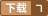### Matlab遗传算法工具箱在非线性优化中的应用-Matlab遗传算法工具箱在非线性优化中的应用.pdf 评分:

Matlab遗传算法工具箱在非线性优化中的应用-Matlab遗传算法工具箱在非线性优化中的应用.pdf 摘　要:投影寻踪是一种降维处理技术,通过它可以将多维分析问题通过投影方向转化为一维问题分析。应用该法的关 键在于寻求最佳投影方向,这可以转化为一个复杂的非线性优化问题来进行解决。选取某地区大气环境质量评价的投影寻踪评价模型,编制相关的目标函数和约束函数,应用基于MATLAB 的遗传算法和直接搜索工具箱进行优化求解。结果表明:该工具箱在求解此类非线性优化问题上的有效性和方便性,从而为各领域应用投影寻踪模型提供了强有力的优化工具。

...展开详情
2019-08-12 上传 大小：258KBMATLAB遗传算法工具箱 立即下载MATLAB遗传算法（工具箱_安装教程_使用实例） 立即下载matlab 遗传算法组合优化实例 立即下载matlab 遗传算法优化BP神经网络程序 立即下载matlab遗传算法geneticbx工具箱和安装步骤和测试代码 立即下载《MATLAB遗传算法工具箱与应用》[雷英杰] 立即下载matlab遗传算法合集 立即下载《Matlab遗传算法工具箱及应用》源码 立即下载MATLAB遗传算法实例 立即下载matlab遗传算法工具箱gatbx 立即下载MATLAB遗传算法电子书合集 立即下载MATLAB和遗传算法 立即下载weixin_39841365